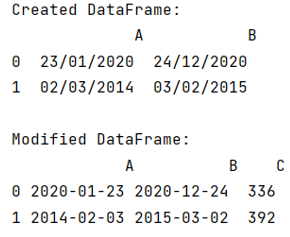# Add column with number of days between dates in DataFrame pandas

Given a Pandas DataFrame, we have to add column with number of days between dates in it.
Submitted by Pranit Sharma, on June 28, 2022

Pandas is a special tool that allows us to perform complex manipulations of data effectively and efficiently. Inside pandas, we mainly deal with a dataset in the form of DataFrame. DataFrames are 2-dimensional data structures in pandas. DataFrames consist of rows, columns, and the data. The Data inside the DataFrame can be of any type.

Pandas is a vast library that consists of almost every kind of mathematical and logical operation. It provides us with a huge amount of methods and functions which makes our data analysis easier. Pandas provide operations for every kind of data type.

## Problem statement

Given a Pandas DataFrame, we have to add column with number of days between dates in it.

## Adding column with number of days between dates in DataFrame pandas

For this purpose, first, change the data types of the columns to dates using the apply() function by passing the pd.to_datetime and then find the number of days between dates and assign it to the new column. To find the differences between two dates and create a new column with the number of days between two dates, we will first have two dates and we will subtract the two dates followed by using the dt.days() method.

Note

To work with pandas, we need to import pandas package first, below is the syntax:

```import pandas as pd
```

Let us understand with the help of an example,

## Python code to add column with number of days between dates in DataFrame pandas

```# Importing pandas package
import pandas as pd

# Creating a dictionary
d = {
'A':['23-01-2020','02-03-2014'],
'B':['24-12-2020','03-02-2015']
}

# Creating dataframe
df = pd.DataFrame(d)

# Display DataFrame
print("Created DataFrame:\n",df,"\n")

# Changing dtypes dates
df[['A','B']] = df[['A','B']].apply(pd.to_datetime)

# Calculating daya
df['C'] = (df['B'] - df['A']).dt.days

# Display modified DataFrame
print("Modified DataFrame:\n",df)
```

### Output

The output of the above program is: